Courses

# Concept Of Atoms And Molecules - Redox Reactions Chemistry Notes | EduRev

## Physical Chemistry

Created by: Asf Institute

## Chemistry : Concept Of Atoms And Molecules - Redox Reactions Chemistry Notes | EduRev

The document Concept Of Atoms And Molecules - Redox Reactions Chemistry Notes | EduRev is a part of the Chemistry Course Physical Chemistry.
All you need of Chemistry at this link: Chemistry

1.  THE CONCEPT OF ATOMS AND MOLECULES

1.1  THE ATOM

The fact that all substances obeyed the laws of chemical combination by weight made the scientists to speculate about the ultimate particles of matter. The most famous of these speculations is due to John Dalton.

Dalton’s Atomic Theory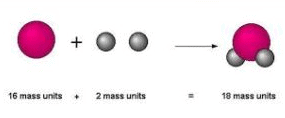Dalton's atomic theory

The main postulates of this atomic theory are

• Matter is discrete (i.e. discontinuous) and is made up of atoms. An atom is the smallest
(chemically) indivisible particle of an element, which can take part in a chemical change.

• Atoms of the same element are identical in all respects, size, shape, structure, etc. and especially weight.

• Atoms of different elements have different properties and different weights.

• Atoms cannot be created or destroyed. So a chemical reaction is nothing but a rearrangement of atoms and the same number of atoms must be present before and after the reaction.

• A compound is formed by the union of atoms of one element with atoms of another in a fixed ratio of small whole numbers (1:1, 1:2, 2:3 etc).

All the postulates of Dalton’s atomic theory have been proved to be incorrect.

An atom is divisible in the sense that it has been proved that atom consists of a number sub atomic particles.

The existence of isotopes for most elements shows that atoms of the same element need not have the same mass. The atomic weight of an element is, in fact, a mean of the atomic masses of the different isotopes of the element.

Parts of atomic mass can be destroyed and an equivalent amount of energy is released during nuclear fission.

Atoms combine in fixed integral ratios, however, there are instances where atoms combine in non-integral ratios e.g. in zinc oxide, and oxygen have not combined in exactly an integral ratio. The atomic ratio of Zn: O= (1+x) : 1, where x is very small fraction. Compounds of this kind are called non-stochiometric compounds or Berthollide compounds as against compounds whose formulae are in accordance with the atomic theory and Proust’s law of definite proportions.

1.2  ATOMIC WEIGHTS

An atom is so minute that it cannot be detected even with the most powerful microscope, let alone placed on a balance pan and weighed. So there is no question of determining the absolute weight of an atom. So chemists decided to determine the relative masses of atoms(i.e. how many times one atom of an element is heavier than another). Hydrogen atom was first selected as standard. i.e.

Atomic weight of an element =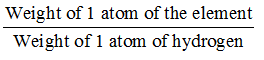When we state that the atomic weight of chlorine is 35.5, we mean that an atom of chlorine is 35.5 times heavier than an atom of hydrogen. It was later felt that the standard for reference for atomic weight may be oxygen, the advantage being that the atomic weights of most other elements became close to whole numbers.

Atomic weight of an element =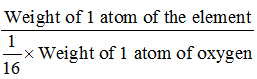The modern reference standard for atomic weights is carbon isotope of mass number 12.

Atomic weight of an element =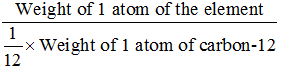On this basis, atomic weight of oxygen 16 was changed to 15.9994.

Nowadays atomic weight is called relative atomic mass and denoted by amu (atomic mass unit). The standard for atomic mass is C12.

• Atomic weight is not a weight but a number.

• Atomic weight is not absolute but relative to the weight of the standard reference element (C12).

• Gram atomic weight is atomic weight expressed in grams, but it has a special significance with reference to a mole.

Dulong and Petit measured the specific heats of a number of metals and found that the product of specific and the atomic weight is a constant, having an approx. value of 6.4.

Specific heat (cal/g – deg)  atomic weight (g/g – atom) ≈ 6.4 (cal/deg.g.atom).

This correlation has been used to ‘correct’ the atomic weights of some elements in the periodic table. Dulong and Petit’s law is applicable only to metals.

1.3  THE MOLECULE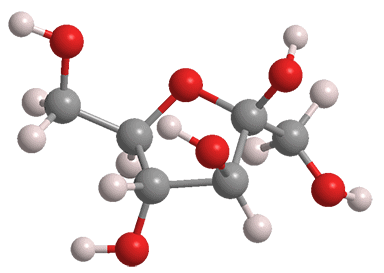Molecule

Avogadro (1811) suggested that the fundamental chemical unit is not an atom but a molecule, which may be a cluster of atoms held together in some manner causing them to exist as a unit. The term molecule means the smallest particle of an element or a compound that can exist free and retain all its properties.

Consider a molecule of sulphur dioxide. It has been established that it contains one atom of sulphur and two atoms of oxygen. This molecule can be split up into atoms of sulphur and oxygen. So the smallest particle of sulphur dioxide that can exist free and retain all its properties is the molecule of sulphur dioxide.

A compound molecule should contain atleast 2 different atoms.The term molecule is also applied to describe the smallest particle of an element which can exist free. Thus a hydrogen molecule is proved to contain 2 atoms; when it is split up into atoms, there will be observed a change in properties

(You may know that nascent hydrogen which may be thought of as atomic hydrogen is a more powerful reducing agent than ordinary hydrogen). Molecules of elementary gases like hydrogen, oxygen, nitrogen, chlorine etc, contain 2 atoms in a molecule; they are diatomic.

Molecules of noble gases like helium, neon, argon, krypton and xenon are monoatomic. Molecules of phosphorus contain 4 atoms (tetratomic) while those of sulphur contain 8 atoms. The number of atoms of an element in a molecule of the element is called its atomicity.

1.4  MOLECULAR WEIGHT

It is the number of times a molecule is heavier than 1/12 of an atom of C-12.

Molecular Weight =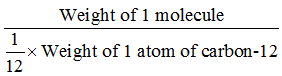• Molecular weight is not a weight but a number.

• Molecular weight is relative and not absolute.

• Molecular weight expressed in grams is called gram-molecular weight.

• Molecular weight is expressed by adding all the atomic weights of all the atoms in a molecule. Thus, the molecular weight of oxygen which contains 2 atoms in a molecule would be (2 x16) = 32. The molecular weight of carbon dioxide, which contains 1 atom of carbon and two atoms of oxygen would be [12+ (2 x16)] = 44. Molecular weight of sulphuric acid, which contains 2 atoms of hydrogen, 1 atom of sulphur and 4 atoms of oxygen is [(2 x1) + (1 x 32) + (4 x16)] = 98.

• Molecular weight is now called relative molecular mass.

1.5  AVOGADRO’S HYPOTHESIS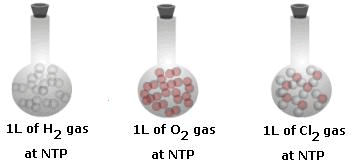Definition of Avogadro's hypothesis

It states that equal volumes of gases at the same temperature and pressure contain equal number of molecules. It means that 1m of hydrogen, oxygen, ammonia, or a mixture of gases taken at the same temperature and pressure contains the same number of molecules.

(a)  To prove that simple elementary gas molecules are diatomic.

Consider the experimental results,

1 volume of hydrogen + 1 molecule of chlorine → 2 volumes of hydrogen chloride at the same temperature and pressure.

1 volume contains ‘n’ molecules. Then n molecules of hydrogen + n molecules of chlorine → 2n molecules of hydrogen chloride.

Cancelling the common ‘n’, we have 1 molecule of hydrogen + 1 molecule of chlorine → 2 molecules of hydrogen chloride.

A molecule of hydrogen chloride should contain atleast 1 atom of hydrogen and 1 atom of chlorine. 2 molecules of hydrogen chloride should contain atleast 2 atoms of hydrogen and 2 atoms of chlorine and these should have come from 1 atoms of hydrogen and 1 atoms of chlorine respectively.

Thus avogadro’s hypothesis enables us to establish that hydrogen and chlorine molecules must contain at least 2 atoms (measurement of the ratio of specific heats of these gases at constant pressure and constant volume, Cp/Cv = 1.4, establishes that these gases are diatomic.

(b) To establish the relationship between molecular weight and vapour density of a gas:

The absolute density of gas is the weight of 1 litre (dm3) of the gas at STP (00C and 1 atmosphere

The relative density or vapour density of a gas =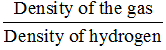Vapour density of a gas =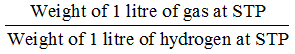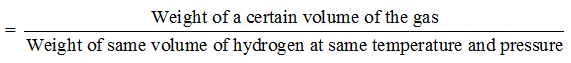So the vapour density of a gas is defined as the ratio of the weight of a certain volume of the gas to the weight of the same volume of hydrogen at the same temperature and pressure.

Vapour density of a gas =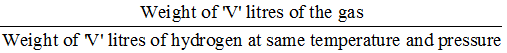Let ‘V’ litres of gas contain ‘n’ molecules.

V.D. of a gas =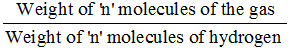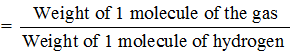V.D. of a gas =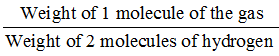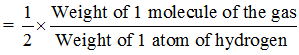V.D. of a gas =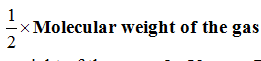Thus, Molecular weight of the gas = 2Vapour Density of the gas.

(a) Gram-molecular volume or Molar volume :

Molecular weight of a gas =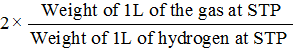Molecular weight of a gas =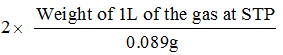Gram-molecular weight of a gas = (2/0.089)weight of 1L of the gas at STP

Gram-molecular weight of a gas = 22.4 weight of 1L of the gas at STP

= weight of 22.4L of the gas at STP.

This establishes that gram-molecular weight of any gas ( or vapour ) occupies the same volume of 22.4L at STP. The volume occupies by a gram-molecular weight of any gas is called molar volume and its value is 22.4 at STP.

Offer running on EduRev: Apply code STAYHOME200 to get INR 200 off on our premium plan EduRev Infinity!

,

,

,

,

,

,

,

,

,

,

,

,

,

,

,

,

,

,

,

,

,

;